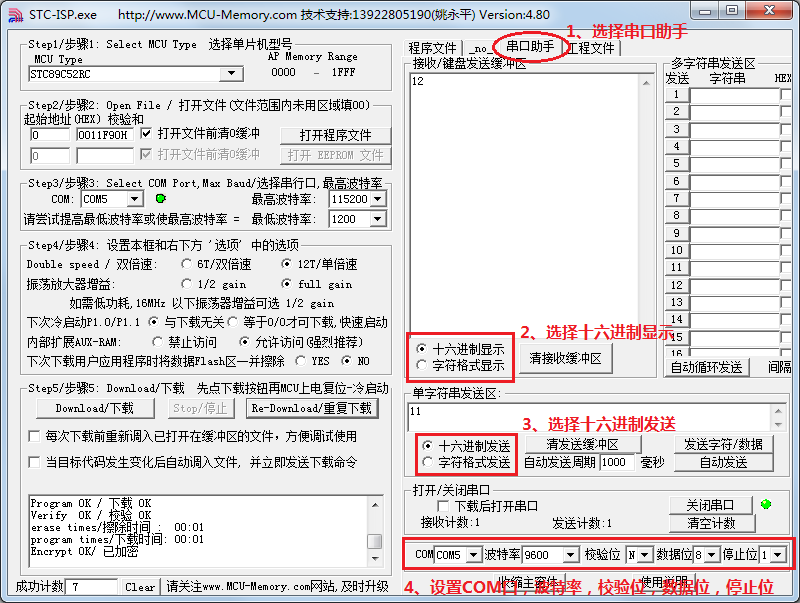# 11.4 单片机 IO 口模拟 UART 串口通信``````#include <reg52.h>
sbit PIN_RXD = P3^0; //接收引脚定义
sbit PIN_TXD = P3^1; //发送引脚定义
bit RxdOrTxd = 0; //指示当前状态为接收还是发送
bit RxdEnd = 0; //接收结束标志
bit TxdEnd = 0; //发送结束标志
unsigned char RxdBuf = 0; //接收缓冲器
unsigned char TxdBuf = 0; //发送缓冲器
void ConfigUART(unsigned int baud);
void StartTXD(unsigned char dat);
void StartRXD();

void main(){
EA = 1; //开总中断
ConfigUART(9600);
while (1){ //配置波特率为9600
while (PIN_RXD); //等待接收引脚出现低电平，即起始位
StartRXD(); //启动接收
while (!RxdEnd); //等待接收完成
StartTXD(RxdBuf+1); //接收到的数据+1后，发送回去
while (!TxdEnd); //等待发送完成
}
}
/* 串口配置函数，baud-通信波特率 */
void ConfigUART(unsigned int baud){
TMOD &= 0xF0; //清零 T0 的控制位
TMOD |= 0x02; //配置 T0 为模式2
TH0 = 256 - (11059200/12)/baud; //计算 T0 重载值
}
/* 启动串行接收 */
void StartRXD(){
TL0 = 256 - ((256-TH0)>>1); //接收启动时的 T0 定时为半个波特率周期
ET0 = 1; //使能 T0 中断
TR0 = 1; //启动 T0
RxdEnd = 0; //清零接收结束标志
RxdOrTxd = 0; //设置当前状态为接收
}
/* 启动串行发送，dat-待发送字节数据 */
void StartTXD(unsigned char dat){
TxdBuf = dat; //待发送数据保存到发送缓冲器
TL0 = TH0; //T0 计数初值为重载值
ET0 = 1; //使能 T0 中断
TR0 = 1; //启动 T0
PIN_TXD = 0; //发送起始位
TxdEnd = 0; //清零发送结束标志
RxdOrTxd = 1; //设置当前状态为发送
}
/* T0 中断服务函数，处理串行发送和接收 */
void InterruptTimer0() interrupt 1{
static unsigned char cnt = 0; //位接收或发送计数
if (RxdOrTxd){ //串行发送处理
cnt++;
if (cnt <= 8){ //低位在先依次发送 8bit 数据位
PIN_TXD = TxdBuf & 0x01;
TxdBuf >>= 1;
}else if (cnt == 9){ //发送停止位
PIN_TXD = 1;
}else{ //发送结束
cnt = 0; //复位 bit 计数器
TR0 = 0; //关闭 T0
TxdEnd = 1; //置发送结束标志
}
}else{ //串行接收处理
if (cnt == 0){ //处理起始位
if (!PIN_RXD){ //起始位为0时，清零接收缓冲器，准备接收数据位
RxdBuf = 0;
cnt++;
}
}else{ //起始位不为0时，中止接收
TR0 = 0; //关闭 T0
}else if (cnt <= 8){ //处理8位数据位
RxdBuf >>= 1; //低位在先，所以将之前接收的位向右移
//接收脚为1时，缓冲器最高位置1，
//而为0时不处理即仍保持移位后的0
if (PIN_RXD){
RxdBuf |= 0x80;
}
cnt++;
}else{ //停止位处理
cnt = 0; //复位 bit 计数器
TR0 = 0; //关闭 T0
if (PIN_RXD){ //停止位为1时，方能认为数据有效
RxdEnd = 1; //置接收结束标志
}
}
}
}``````